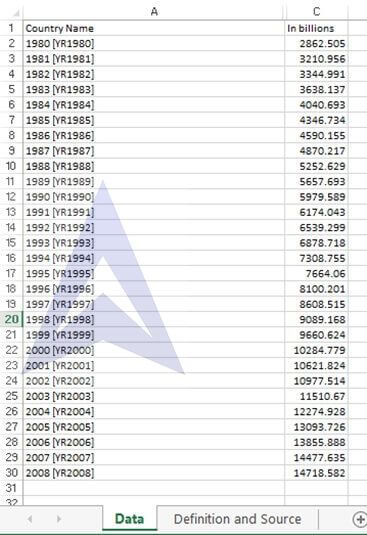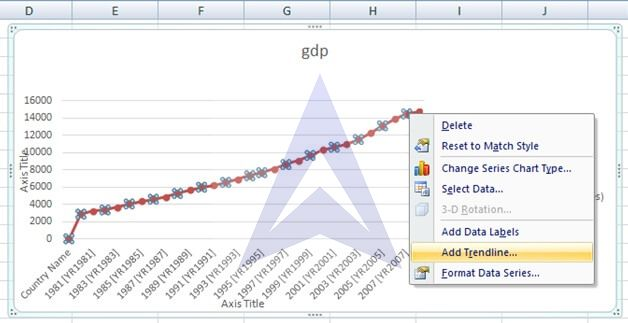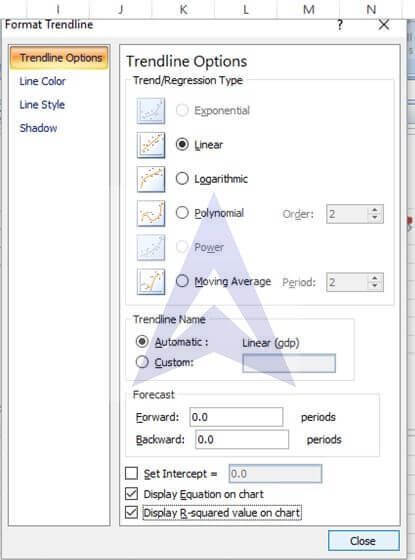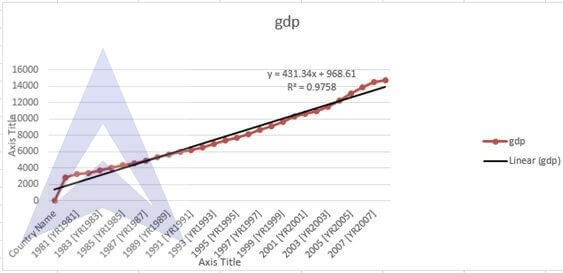# Time Series Analysis: Plotting Linear Trend and Forecasting

Gross domestic product (GDP) is a major indicator of a nation’s overall economic activity. It consist of personal consumption expenditures, gross domestic investment, net experts of goods and services, and government consumption expenditures. The GDP (in billions of current dollars) for the United States from 1980 to 2008 is stored in GDP.

## A. Plot the data. Compute a linear trend forecasting equation and plot the trend line

How to get GDP data:

For data on US GDP we can download the data from World Bank’s World Development Indicators database. Choose the series as GDP at market price (constant US\$ 2010) > select Unites States as Country> Choose the required time period (1980-2008). Download the data in excel format.Step 2

Plot a line graph. Click on insert and select icon of line graph. Select data for y axis from B1 to B30 while for x axis select data from A1 to A30. To plot a trendline select the data points right click and you will find the option of adding a trendline selct the option. Then right click on trendline and select the option of formatting trendline select display equation and R^2. Click ok. The graph with all the iformation will be plotted.The forecasting trend equation is

Y=431.34*x+968.61
GDP=431.34*(Year) + 968.61

## B. What are your forecasts for 2009 to 2010?

```{`
X=2009 (30th observation)
Y=431.34*30+968.61
Y=13909.01

X=2010 (31st observation)
Y=431.34*(31)+968.61
Y= 14340.35
`}```

The GDP in 2009 should be 13909 billion dollars while in 2010 it should be 14340 billion dollars.

### C. What conclusions can you reach concerning the trend in GDP?

The US GDP data from 1980-2008 shows a linear trend and every year. Every year the GDP increases by 431.34 billion dollars on average at constant prices of 2010 US \$.

## Excel Econometrics Tutorials

Multiple Regression Analysis

Time Series Analysis

Data Analysis